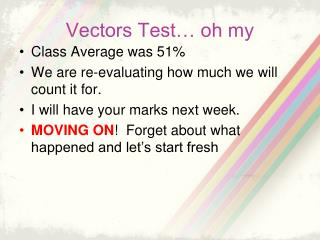DownloadDownload PresentationVectors Test… oh my

# Vectors Test… oh my

Download Presentation## Vectors Test… oh my

- - - - - - - - - - - - - - - - - - - - - - - - - - - E N D - - - - - - - - - - - - - - - - - - - - - - - - - - -
##### Presentation Transcript

1. Vectors Test… oh my • Class Average was 51% • We are re-evaluating how much we will count it for. • I will have your marks next week. • MOVING ON! Forget about what happened and let’s start fresh

2. TRIGONOMETRY Trig Ratios of Right Angle Triangles Section 5.1

3. Review… The acute angles of a right angle triangle are complementary, which means: angleA + angleB = 90˚ A c b C B a

4. Review… Side c is called the hypotenuse A c b C B a

5. Review… SOH CAH TOA!!!!! cosA = adjacent = b hypotenuse c A c b C B a

6. Review… SOH CAH TOA!!!!! sinA = opposite = a hypotenuse c A c b C B a

7. Review… SOH CAH TOA!!!!! tanA = opposite = a adjacent b A c b C B a

8. 3 New Ratios Secant (sec), cosecant (csc) and cotangent (cot) We can get these ratios by inverting the three ratios that we already know… A c b C B a

9. Secant is the inverse of cosine. secA = 1 = hypotenuse = c cosA adjacent b A c b C B a

10. Cosecant is the inverse of sine. cscA = 1 = hypotenuse = c sinA opposite a A c b C B a

11. Cotangent is the inverse of tangent. cotA = 1 = adjacent = b tanA opposite a A c b C B a

12. Example 1 • Find all sinA, cosA, tanA, secA, cscA, tanA First, you need to findthe hypotenuse! a2 + b2 = c2 32 + 42 = c2 c = 5 A 5 3 C B 4

13. Example 1 Find ratios sinA, cosA, tanA, secA, cscA, tanA sinA = opp = 4 hyp 5 A 5 3 C B 4

14. Example 1 Find ratios sinA, cosA, tanA, secA, cscA, tanA cosA = adj = 3 hyp 5 A 5 3 C B 4

15. Example 1 Find ratios sinA, cosA, tanA, secA, cscA, tanA tanA = opp = 4 adj 3 A 5 3 C B 4

16. Example 1 Find ratios sinA, cosA, tanA, secA, cscA, tanA secA = hyp = 5 adj 3 A 5 3 C B 4

17. Example 1 Find ratios sinA, cosA, tanA, secA, cscA, tanA cscA = hyp = 5 opp 4 A 5 3 C B 4

18. Example 1 Find ratios sinA, cosA, tanA, secA, cscA, tanA cotA = adj = 3 opp 4 A 5 3 C B 4

19. Another Useful Ratio… tanA = sinA cosA And so… cotA = cosA sinA

20. Remarkable Angles • Look at workbook p.191-192. Take 10 minutes to do Activity 2. THERE WILL BE MORE NOTES so do not change spots.

21. Remarkable Angles Notice that for - 0˚ and 90˚, sine and cosine are opposite - 30˚ and 60˚, sine and cosine are opposite

22. Example 2 Using the chart, find the exact values of x and y sin30˚= 1 2 1 = opp 2 hyp 1 = x 2 10 x = 5 A 10 x 30˚ C y B Cross Multiplyand simply

23. Example 2 Using the chart, find the exact values of x and y cos30˚= √3 2 √3 = adj 2 hyp √3 = y 2 10 x = 5√3 A 10 x 30˚ C y B Cross Multiplyand simply

24. HOMEWORK Workbook p.191 #1a, 2 (solve means find all sides and all angles) p.193 #3,4